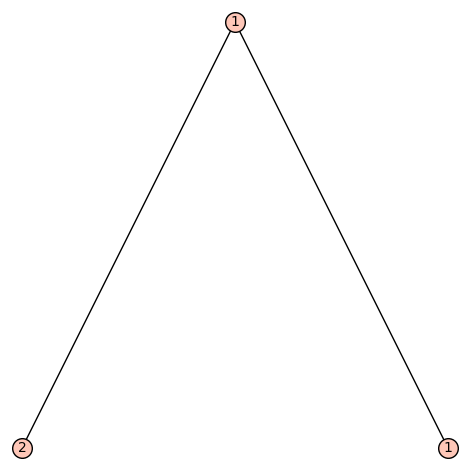Ask Your Question

# Revision history [back]

This (kind of silly) limitation is documented as a Warning.

Since you can use arbitrary objects as labels, here's a way around it:

class Wrap(object):
def __init__(self, obj):
self.obj = obj
def __repr__(self):
return repr(self.obj)

n2 = LabelledOrderedTree([], label=Wrap(2))
n1 = LabelledOrderedTree([], label=Wrap(1))
root = LabelledOrderedTree([n2, n1], label=Wrap(1))
root.plot()


The two instances Wrap(1) and Wrap(1) are different Python objects, but they look the same, so it works.

This (kind of silly) limitation is documented as a Warning.

Since you can use arbitrary objects as labels, here's a way around it:

class Wrap(object):
def __init__(self, obj):
self.obj = obj
def __repr__(self):
return repr(self.obj)

n2 = LabelledOrderedTree([], label=Wrap(2))
n1 = LabelledOrderedTree([], label=Wrap(1))
root = LabelledOrderedTree([n2, n1], label=Wrap(1))
root.plot()The two instances Wrap(1) and Wrap(1) are different Python objects, but they look the same, so it works.

Alternatively, if you want new distinct labels:

sage: root.canonical_labelling().plot()